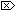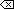The Open Group Base Specifications Issue 6
IEEE Std 1003.1-2001

#### NAME

exp2, exp2f, exp2l - exponential base 2 functions

#### SYNOPSIS

```#include <math.h> double exp2(double x); float exp2f(float x); long double exp2l(long double x); ```

#### DESCRIPTION

[CX]The functionality described on this reference page is aligned with the ISO C standard. Any conflict between the requirements described here and the ISO C standard is unintentional. This volume of IEEE Std 1003.1-2001 defers to the ISO C standard.These functions shall compute the base-2 exponential of x.

An application wishing to check for error situations should set errno to zero and call feclearexcept(FE_ALL_EXCEPT) before calling these functions. On return, if errno is non-zero or fetestexcept(FE_INVALID | FE_DIVBYZERO | FE_OVERFLOW | FE_UNDERFLOW) is non-zero, an error has occurred.

#### RETURN VALUE

Upon successful completion, these functions shall return 2x.

If the correct value would cause overflow, a range error shall occur and exp2(), exp2f(), and exp2l() shall return the value of the macro HUGE_VAL, HUGE_VALF, and HUGE_VALL, respectively.

If the correct value would cause underflow, and is not representable, a range error may occur, and [MX]either 0.0 (if supported), oran implementation-defined value shall be returned.

[MX]If x is NaN, a NaN shall be returned.

If x is ±0, 1 shall be returned.

If x is -Inf, +0 shall be returned.

If x is +Inf, x shall be returned.

If the correct value would cause underflow, and is representable, a range error may occur and the correct value shall be returned.#### ERRORS

These functions shall fail if:

Range Error
The result overflows.

If the integer expression (math_errhandling & MATH_ERRNO) is non-zero, then errno shall be set to [ERANGE]. If the integer expression (math_errhandling & MATH_ERREXCEPT) is non-zero, then the overflow floating-point exception shall be raised.

These functions may fail if:

Range Error
The result underflows.

If the integer expression (math_errhandling & MATH_ERRNO) is non-zero, then errno shall be set to [ERANGE]. If the integer expression (math_errhandling & MATH_ERREXCEPT) is non-zero, then the underflow floating-point exception shall be raised.

The following sections are informative.

None.

#### APPLICATION USAGE

For IEEE Std 754-1985 double, 1024 <= x implies exp2( x) has overflowed. The value x < -1022 implies exp( x) has underflowed.

On error, the expressions (math_errhandling & MATH_ERRNO) and (math_errhandling & MATH_ERREXCEPT) are independent of each other, but at least one of them must be non-zero.

None.

None.